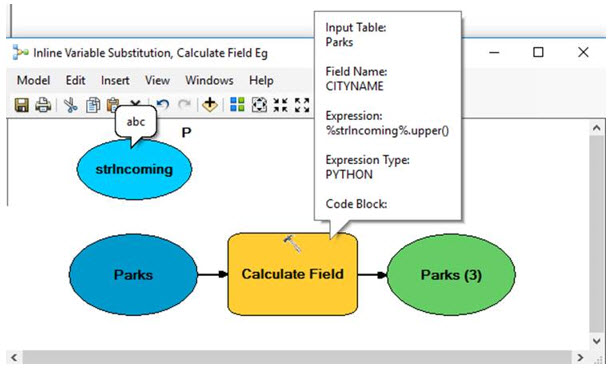PROBLEM

# The Calculate Field tool expression does not evaluate model variables of type string using the expected inline variable substitution convention

Last Published: April 25, 2020

## Description

When using the Calculate Field tool in a model, if the Calculate Field expression requires a model variable of type string. That model variable must be enclosed in quotes. For example, the following Calculate Field tool expressions convert a model variable (of type string) to uppercase:

```VB:     	UCase("%StratumName%")
... and not     UCase(%StratumName%)
```
```Python :	("%StratumName%").upper()
... and not 	(%StratumName%).upper()  ```

Without the quotes, the model fails. The illustration below shows an example model with relevant parameters:## Cause

The VBScript and Python expressions fail because the model convention of denoting model variables with surrounding '%' signs is not recognized in the model builder manner by the Calculate Field tool expression parser (the Python interpreter or the VBScript interpreter).

Given the above model illustration, there is:

• a string variable named strIncoming (which has a value of 'abc')

• a Calculate Field expression of %strIncoming%.upper()

Consequently, the parser searches for a variable named 'abc' and that variable's value to convert to uppercase. However, because it is unable to find the variable named 'abc', the model fails with an error results.

## Solution or Workaround

Modify the Calculate Field expression to enclose the string variable in quotes, for example :

`VB: UCase("%StratumName%")`
`Python: ("%StratumName%").upper()`

Article ID:000017185

Software:
• ArcMap

Get help from ArcGIS experts

Contact technical support